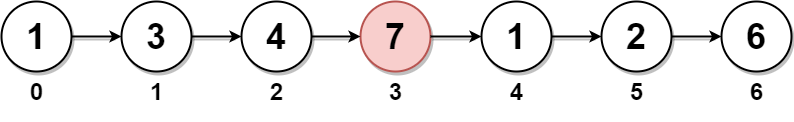# 2095. Delete the Middle Node of a Linked List | LeetCode

## 2095. Delete the Middle Node of a Linked List

You are given the `head` of a linked list. Delete the middle node, and return the `head` of the modified linked list.

The middle node of a linked list of size `n` is the `⌊n / 2⌋th` node from the start using 0-based indexing, where `⌊x⌋` denotes the largest integer less than or equal to `x`.

• For `n` = `1``2``3``4`, and `5`, the middle nodes are `0``1``1``2`, and `2`, respectively.

Example 1:```Input: head = [1,3,4,7,1,2,6]
Output: [1,3,4,1,2,6]
Explanation:
The above figure represents the given linked list. The indices of the nodes are written below.
Since n = 7, node 3 with value 7 is the middle node, which is marked in red.
We return the new list after removing this node.
```

Example 2:```Input: head = [1,2,3,4]
Output: [1,2,4]
Explanation:
The above figure represents the given linked list.
For n = 4, node 2 with value 3 is the middle node, which is marked in red.
```

Example 3:```Input: head = [2,1]
Output: 
Explanation:
The above figure represents the given linked list.
For n = 2, node 1 with value 1 is the middle node, which is marked in red.
Node 0 with value 2 is the only node remaining after removing node 1.```

Constraints:

• The number of nodes in the list is in the range `[1, 105]`.
• `1 <= Node.val <= 105`